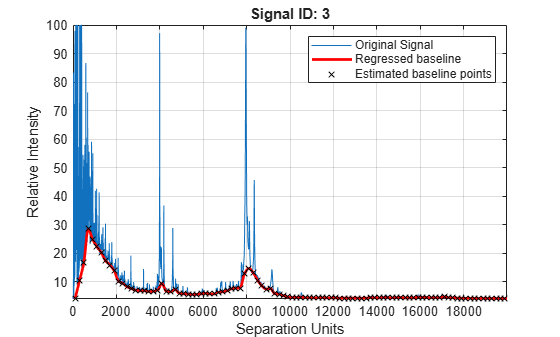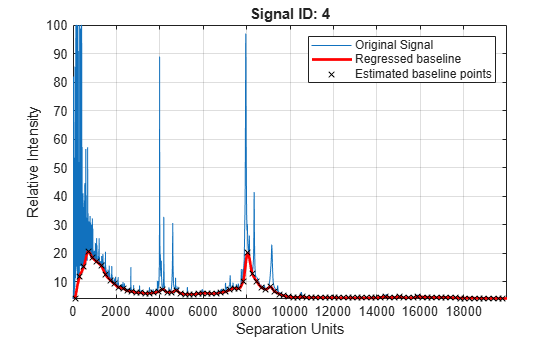Main Content

# msbackadj

Correct baseline of signal with peaks

## Syntax

``yOut = msbackadj(X,Intensities)``
``yOut = msbackadj(X,Intensities,Name,Value)``

## Description

example

````yOut = msbackadj(X,Intensities)` adjusts the variable baseline of a raw signal with peaks by performing the following steps. Estimate the baseline within multiple shifted windows of width 200 separation units.Regress the varying baseline to the window points using a spline approximation.Adjust the baseline of the peak signals supplied by the input `Intensities`.Return the adjusted intensity values in the output matrix `yOut`. ```

example

````yOut = msbackadj(X,Intensities,Name,Value)` sets additional options specified by one or more name-value pair arguments. For example, `msbackadj(X,Intensities,'WindowSize',300)` sets the width of the shifting window to 300 separation units.```

## Examples

collapse all

Load a sample mass spec data including `MZ_lo_res`, a vector of m/z values, and `Y_lo_res`, a matrix of intensity values.

`load sample_lo_res`

Adjust the baseline of a group of spectrograms and show only the third spectrum and its estimated background.

`YB = msbackadj(MZ_lo_res,Y_lo_res,'ShowPlot',3);`Estimate the baseline for every spectrum in `Y_lo_res` using an anonymous function to describe an m/z dependent parameter. Then plot the estimated background for the fourth spectrum.

```wf = @(mz) 200 + .001 .* mz; msbackadj(MZ_lo_res,Y_lo_res,'StepSize',wf,'ShowPlot',4);```## Input Arguments

collapse all

Separation-unit values for a set of signals with peaks, specified as a vector.

The number of elements in the vector equals the number of rows in `Intensities`. The separation unit can quantify wavelength, frequency, distance, time, or m/z ratio depending on the instrument that generates the signal data.

Data Types: `double`

Intensity values for a set of peaks that share separation-unit range, specified as a numeric matrix.

Each row corresponds to a separation-unit value, and each column corresponds to either a set of signals with peaks or a retention time. The number of rows equals the number of elements in `X`. The signal data can come from any separation technique, such as spectroscopy, NMR, electrophoresis, chromatography, or mass spectrometry.

Data Types: `double`

### Name-Value Pair Arguments

Specify optional comma-separated pairs of `Name,Value` arguments. `Name` is the argument name and `Value` is the corresponding value. `Name` must appear inside quotes. You can specify several name and value pair arguments in any order as `Name1,Value1,...,NameN,ValueN`.

Example:

Shifting window size, specified as a positive scalar or function handle. By default, `msbackadj` estimates baseline points for windows with a width of 200 separation units.

If you specify a function handle, the function is evaluated at the respective `X` values and returns a variable width for the window. Specifying a function handle is useful when the resolution of the signal is dissimilar at different regions.

The result of `msbackadj` depends on the window size and step size. Define the parameters based on the width of your peaks in the signal and the presence of possible drifts. If you have wider peaks towards the end of the signal, consider using variable window sizes and/or step sizes.

Example: `'WindowSize',300`

Data Types: `double` | `function_handle`

Step size for the shifting window, specified as a positive scalar or function handle. By default, `msbackadj` estimates baseline points for windows placed every 200 separation units.

If you specify a function handle, the function is evaluated at the respective separation-unit values and returns the distance between adjacent windows.

Example: `'StepSize',150`

Data Types: `double` | `function_handle`

Method to regress the window estimated points to a soft curve, specified as one of the following:

• `'pchip'` — Shape-preserving piecewise cubic interpolation. The interpolated value at a query point is based on a shape-preserving piecewise cubic interpolation of the values at neighboring grid points.

• `'linear'` — Linear interpolation. The interpolated value at a query point is based on linear interpolation of the values at neighboring grid points in each respective dimension.

• `'spline'` — Spline interpolation. The interpolated value at a query point is based on a cubic interpolation of the values at neighboring grid points in each respective dimension.

Example: `'RegressionMethod','linear'`

Data Types: `char` | `string`

Method to find likely baseline (background) value in every window, specified as one of the following:

• `'quantile'` — Quantile value is set to 10%.

• `'em'` — Every sample is the independent and identically distributed (i.i.d) draw of any of two normal distributed classes (background or peaks). Because the class label is hidden, the distributions are estimated with an Expectation-Maximization algorithm. The ultimate baseline value is the mean of the background class.

Example: `'EstimationMethod','em'`

Data Types: `char` | `string`

Method to smooth the curve of estimated points, specified as one of the following:

• `'none'` — No smoothing.

• `'lowess'` — Linear fit.

• `'loess'` — Quadratic fit.

• `'rlowess'` — Robust linear fit.

• `'rloess'` — Robust quadratic fit.

Example: `'SmoothMethod','lowess'`

Data Types: `char` | `string`

Quantile value, specified as a positive scalar between `0` and `1`.

Example: `'QuantileValue',0.2`

Data Types: `double`

Flag to preserve the height of the tallest peak in the signal, specified as `true` or `false`. By default, peak heights are not preserved.

Example: `'PreserveHeights',true`

Data Types: `logical`

Flag to plot the regressed baseline, original signal, and estimated baseline points, specified as `true`, `false`, or a positive integer.

The default behavior is as follows:

• When you call `msbackadj` without an output argument, the plot is shown. Only the first signal from the input `Intensities` is plotted.

• When you call `msbackadj` with an output argument, the plot is not shown. But you can get the plot by also setting `'ShowPlot'` to `true`.

You can also specify an index to one of the signals (columns) in `Intensities` to show the corresponding plot of that signal.

Example: `'ShowPlot',5`

Data Types: `double` | `logical`

## Output Arguments

collapse all

Adjusted intensity values, returned as a matrix.

Introduced before R2006a

## Support평가판 신청